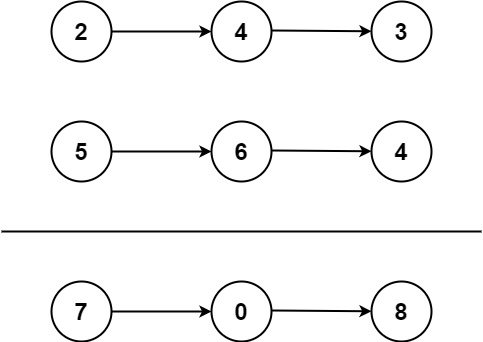Amazon

Apple

Bloomberg

Coupang

Microsoft

Paypal

Uber

VMware

Yahoo

2021-01-21

## 2. Add Two Numbers

### Question:

You are given two non-empty linked lists representing two non-negative integers. The digits are stored in reverse order, and each of their nodes contains a single digit. Add the two numbers and return the sum as a linked list.

You may assume the two numbers do not contain any leading zero, except the number 0 itself.

#### Example 1:``````Input: l1 = [2,4,3], l2 = [5,6,4]
Output: [7,0,8]
Explanation: 342 + 465 = 807.
``````

#### Example 2:

``````Input: l1 = , l2 = 
Output: 
``````

#### Example 3:

``````Input: l1 = [9,9,9,9,9,9,9], l2 = [9,9,9,9]
Output: [8,9,9,9,0,0,0,1]
``````

Constraints:

• The number of nodes in each linked list is in the range `[1, 100]`.
• `0 <= Node.val <= 9`
• It is guaranteed that the list represents a number that does not have leading zeros.

### Solution:

Traverse two linkedlist at the same time and check if there’s a value for the next digit.

``````/**
* Definition for singly-linked list.
* public class ListNode {
*     int val;
*     ListNode next;
*     ListNode() {}
*     ListNode(int val) { this.val = val; }
*     ListNode(int val, ListNode next) { this.val = val; this.next = next; }
* }
*/
class Solution {
public ListNode addTwoNumbers(ListNode l1, ListNode l2) {
ListNode result = new ListNode();
ListNode curr = result;
int next = 0;
while (l1 != null || l2 != null) {
curr.next = new ListNode();
curr = curr.next;
int total = 0;
if (l1 != null) {
next += l1.val;
l1 = l1.next;
}
if (l2 != null) {
next += l2.val;
l2 = l2.next;
}
curr.val = next % 10;
next = next / 10;
}
if (next == 1) {
curr.next = new ListNode();
curr.next.val = 1;
}
return result.next;
}
}
``````